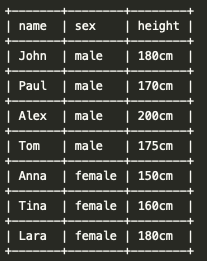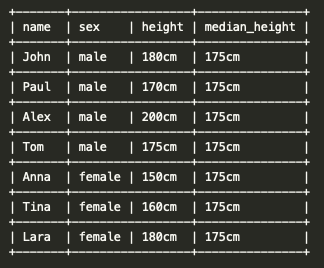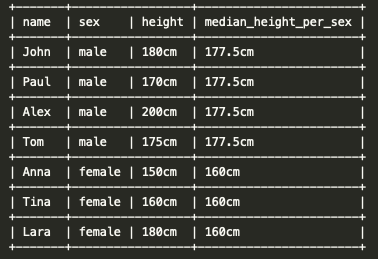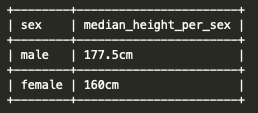# Can I calculate a median using GROUP BY in BigQuery?

This is a very short and sweet post on how to calculate the median for different subgroups of data that you want to aggregate like when using GROUP BY

Hello! In case you’re just looking for the solution and not a long-winded explanation, here is an example for how to do it:

# The Solution

``````SELECT
DISTINCT sex,
median_height_per_sex
FROM (
SELECT
name,
sex,
height,
PERCENTILE_CONT(height, 0.5) OVER(PARTITION BY sex)
AS median_height_per_sex
FROM
height_data)
``````

# Background on the problem

When we want to calculate an aggregate statistic for different subgroups of data, we normally use GROUP BY and an already implemented function to compute the desired function over the field we select. For example, if we wanted to calculate the average height per sex from a table named `height_data`, we simply do:

``````SELECT
sex,
AVG(height) AS average_height
FROM
height_data
GROUP BY
sex
``````

This query will return one row per sex and its corresponding average height. In other words, `AVG` computes one value for each group defined by the GROUP BY function. Functions that like `AVG` return one value per group of rows (such as `MAX`, `MIN`, `SUM`, etc) are called aggregate functions.

After a Google search you can find out that to calculate the median in BigQuery you have to use `PERCENTILE_CONT(x, 0.5)` where x is the field we want to calculate the median over and 0.5 indicates the 50th percentile. If you don’t read any further, what you would try to do is compute the median per sex using `SELECT sex, PERCENTILE_CONT(height, 0.5) as median_height FROM height_data GROUP BY sex`. However, this doesn’t work!

# What differentiates `PERCENTILE_CONT` from `AVG`?

Well, `PERCENTILE_CONT` isn’t an aggregate function but an analytical function. An analytical function (sometimes also called more explicitly non-aggregate function) calculates one value per row rather than per group of rows. Practically, this means `PERCENTILE_CONT` can’t be applied at the same time as GROUP BY like `AVG`.

The syntax to apply `PERCENTILE_CONT` is also a bit different:

``````SELECT
name,
sex,
height,
PERCENTILE_CONT(height, 0.5) OVER() AS median_height
FROM
height_data
``````

The OVER() clause defines the subgroup over which the median will be calculated. If left empty as above, the median will be calculated for all the rows and assigned to all of them. If our height_data table looks something like this:The result of applying the query above will be:Remember the median is the value that is the middle position after ordering them in magnitude. In this case, the ordered heights would be [150, 160, 170, 175, 180, 180, 200] and the height in the middle (the median_height) is 175. This is assigned to every row in the table. However, this is not exactly what we want. Two things are missing:

## 1) We want to calculate one median_height per sex.

As mentioned earlier, the `OVER()` clause is used to define the different subgroups over which the median will be calculated. When we want to specify the subgroups, we use `PARTITION BY` inside of the parenthesis followed by the fields we want to use to separate the subgroups. In our case, this means:

``````SELECT
name,
sex,
height,
PERCENTILE_CONT(height, 0.5) OVER(PARTITION BY sex)
AS median_height_per_sex
FROM
height_data
``````

The results of the query will be:When we have an even number of rows, the median is calculated by linear interpolation between the two middle values. For the case of `male`, we have [170, 175, 180, 200]; the two middle values are 175 and 180, the middle point between those values is 177.5. Therefore, the median for `male` rows is 177.5. The median for the `female` rows ([150, 160, 180]) is 160.

Almost there! We only need to merge all of the male and female rows into a single one.

## 2) We want to have as a result only one row per sex, not one row per person.

To do so, we just need to see that if we remove the `name` and `height` fields, all `male` rows are the same. The same happens for `female` rows. Therefore, we can simply select the distinct rows based on `sex` and `median_height_per_sex` as follows:

``````SELECT
DISTINCT sex,
median_height_per_sex
FROM (
SELECT
name,
sex,
height,
PERCENTILE_CONT(height, 0.5) OVER(PARTITION BY sex)
AS median_height_per_sex
FROM
height_data)
``````

This is equivalent to doing:

``````SELECT
sex,
median_height_per_sex
FROM (
SELECT
name,
sex,
height,
PERCENTILE_CONT(height, 0.5) OVER(PARTITION BY sex)
AS median_height_per_sex
FROM
height_data) GROUP BY sex, median_height_per_sex
``````

The result of these queries is as follows:which is what we wanted!

Follow me on Twitter @iamhectorotero for more interesting posts and content about data-related topics!

Written on May 17, 2020